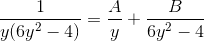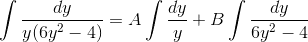# Differential Equation - Bernoulli Equation

pat666

## Homework Statement

solve the differential equation
$$y'(t)=-4y+6y^3$$

## The Attempt at a Solution

I'm pretty sure (not positive) that this is a Bernoulli Equation.
I've been following this wiki in an attempt to solve: http://en.wikipedia.org/wiki/Bernoulli_differential_equation
$$(y'(t)=-4y+6y^3)/y^3$$
$$y'(t)/y^3=-4/y^2+6$$
$$w=1/y^2$$
$$y'(t)*w/y=-4w+6$$

I'm stuck here, also theres no x's or t's here so im not sure that it is a Bernoulli Equation?

thanks for any help

## Answers and Replies

SVXX
dy/dt = 6y^3 - 4y. This is a variable separable differential equation, no Bernoulli pangs required. dy/(y)(6y^2 - 4) = dt. Integrate the LHS preferably using partial fractions and voila.

pat666
$$dy/dt=-4y+6y^3$$
$$dy/(y(-4+6y^2))=dt$$
$$u=y^2, du =2y dy$$
$$=1/2 \int 1/(u(6u-4))$$
etc etc etc
$$t=1/8 (ln(3y^2-2)-2ln(y))+c$$
$$8t-c=ln((3y^2-2)/(y^2))$$
$$ce^t=(3y^2-2)/y^2$$

I am again stuck, how do I get y=----- out of this??

Thanks

SVXX
Mate, you need to use partial fractions to separate the denominator and integrate on each term separately.Find A and B using the concept of partial fractions (this page is pretty neat...teaches you everything you need to know about them : http://www.math.ucdavis.edu/~kouba/CalcTwoDIRECTORY/partialfracdirectory/PartialFrac.html), then multiply dy on both sides of the equation and integrate term by term.Homework Helper
$$dy/dt=-4y+6y^3$$
$$dy/(y(-4+6y^2))=dt$$
$$u=y^2, du =2y dy$$
$$=1/2 \int 1/(u(6u-4))$$
etc etc etc
$$t=1/8 (ln(3y^2-2)-2ln(y))+c$$
$$8t-c=ln((3y^2-2)/(y^2))$$
$$ce^t=(3y^2-2)/y^2$$

I am again stuck, how do I get y=----- out of this??

Thanks

From
$$ce^t= \frac{3y^2- 2}{y^2}$$
multiply both sides by $y^2$:
$$ce^ty^2= 3y^2- 2$$

$$3y^2- ce^ty^2= 2$$
$$(3- ce^t)y^2= 2$$

Can you finish that?

(Does the problem require that you solve for x? Solutions to first order equations are often left as implicit functions.)

pat666
certainly can:
$$y=sqrt(2/((3-ce^t)))$$
I think thats right? and it keeps me away from partial fractions which I forgot about 3yrs ago.

Thanks svxx and Hallsofivy

edit:

oops, I used partial fractions in the integrations, guess I remember procedures and not names of said procedures...

Last edited: